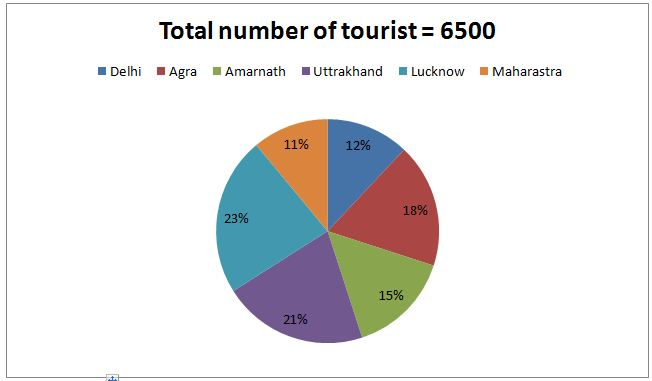# LIC AAO/SBI PO Prelims Quantitative Aptitude Questions 2019 (Day-30)

Dear Aspirants, Our IBPS Guide team is providing new series of Quantitative Aptitude Questions for LIC AAO/SBI PO 2019 so the aspirants can practice it on a daily basis. These questions are framed by our skilled experts after understanding your needs thoroughly. Aspirants can practice these new series questions daily to familiarize with the exact exam pattern and make your preparation effective.

[WpProQuiz 6060]

### Click Here for SBI PO Pre 2019 High-Quality Mocks Exactly on SBI Standard

Directions (Q. 1 – 5): What approximate value should come in place of (?) in the following questions?

1) ? × 5 = (1564.81 + 799.78) ÷ 11 + (25.92)2 + (2/7) of 846

a) 318

b) 356

c) 434

d) 227

e) 295

2) (4/9) of (62/13) of 170 = 13 % of 799 + (2/5) of (?)

a) 650

b) 720

c) 780

d) 630

e) 560

3) (3564 ÷ 9.12) ÷ 3 × 11 + 13% of 499 – 17 % of 800.23 =?

a) 1452

b) 1627

c) 1381

d) 1575

e) 1293

4) 4913 – ? ÷ √145 + (3/7) of 384 – 42 = 22

a) 1528

b) 1416

c) 1632

d) 1344

e) 1750

5) 56 % of 4249 + 12 % of ? = 2787.79 + (15.11)2

a) 4500

b) 3725

c) 6050

d) 5275

e) 5800

Directions (6 – 10): Study the following information carefully and answer the given questions:

The following pie chart shows the percentage distribution of total number of tourist went for a 6 different cities and the table shows the male and female tourist among them.Cities Male : Female Delhi 6 : 7 Agra 5 : 4 Amarnath 8 : 7 Uttrakhand 7 : 8 Lucknow 2 : 3 Maharashtra 4 : 1

6) Find the difference between the total number of male tourist went to Delhi and Amarnath together to that of total number of female tourist went to Agra and Lucknow together?

a) 428

b) 453

c) 537

d) 512

e) None of these

7) If 50 % of male tourist went to Amarnath and Lucknow together is 30 years less than the age and 30 % of female tourist went to Delhi and Agra together is 30 years less than the age, then find the total number of tourist went to above mentioned places are less than the age of 30?

a) 841

b) 759

c) 872

d) 766

e) None of these

8) If 75 % of male tourist went to Delhi and Maharashtra together are private employees and 25 % of female tourist went to Agra and Uttrakhand together are government employees, then find the ratio between the total number of male government employees in Delhi and Maharashtra together to that of total number of female private employees in Agra and Uttrakhand together?

a) 132: 755

b) 356: 859

c) 75: 187

d) 233: 936

e) None of these

9) Total number of tourist went to Delhi is what percentage of total number of tourist went to Amarnath?

a) 65 %

b) 80 %

c) 70 %

d) 75 %

e) None of these

10) Find the total number of male tourist went to all the given cities together?

a) 3568

b) 3216

c) 3337

d) 3789

e) None of these

Directions (1-5):

5x = (1565 + 800) ÷ 11 + (26)2 + (2/7)*847

5x = (2365/11) + 676 + 242

5x = 215 + 676 + 242

5x = 1133

X = 1133/5

X = 227

(4/9)*(63/13)*169 = 13 % of 800 + (2/5)*x

364 = (13/100)*800 = (2x/5)

364 – 104 = (2x/5)

260*(5/2) = x

x = 650

(3564/9)/3 × 11 + (13/100)*500 – (17/100)*800 = x

1452 + 65 – 136 = x

x = 1381

17 – (x/12) + (3/7)*385 – 42 = 22

17 – (x/12) + 165 – 42 = 22

17 + 165 – 42 – 22 = (x/12)

118 = (x/12)

X = 118*12 = 1416

56 % of 4250 + 12 % of x = 2788 + 152

(56/100)*4250 + (12/100)*x = 2788 + 225

(12/100)*x = 2788 + 225 – 2380

(12/100)*x = 633

X = 633*(100/12) = 5275

Directions (6-10):

The total number of male tourist went to Delhi and Amarnath together

= > 6500*(12/100)*(6/13) + 6500*(15/100)*(8/15)

= > 360 + 520 = 880

The total number of female tourist went to Agra and Lucknow together

= > 6500*(18/100)*(4/9) + 6500*(23/100)*(3/5)

= > 520 + 897 = 1417

Required difference = 1417 – 880 = 537

The total number of male tourist of 30 years less than the age went to Amarnath and Lucknow together

= > [6500*(15/100)*(8/15) + 6500*(23/100)*(2/5)]*(50/100)

= > [520 + 598]*(1/2)

= > 559

The total number of female tourist of 30 years less than the age went to Delhi and Agra together

= > [6500*(12/100)*(7/13) + 6500*(18/100)*(4/9)]*(30/100)

= > [420 + 520]*(30/100)

= > 282

Required total = 559 + 282 = 841

The total number of male government employees went to Delhi and Maharashtra together

= > [6500*(12/100)*(6/13) + 6500*(11/100)*(4/5)]*(25/100)

= > [360 + 572]*(1/4)

= > 233

The total number of female private employees went to Agra and Uttrakhand together

= > [6500*(18/100)*(4/9) + 6500*(21/100)*(8/15)]*(75/100)

= > [520 + 728]*(3/4)

= > 936

Required ratio = 233: 936

Required % = (12/15)*100 = 80 %

The total number of male tourist went to all the given cities together

= > [6500*(12/100)*(6/13) + 6500*(18/100)*(5/9) + 6500*(15/100)*(8/15) + 6500*(21/100)*(7/15) + 6500*(23/100)*(2/5) + 6500*(11/100)*(4/5)]

= > [360 + 650 + 520 + 637 + 598 + 572]

= > 3337